# More on Half-Planes

Go back to  'Linear-Equations'

Let us understand in more detail how linear inequations correspond to half-planes. First, consider an arbitrary linear equation, which we can always write as follows:

$y=mx+b\;\;\;\;\;\;\;\;\;(how?)$

Suppose that the graph of this equation is the following line: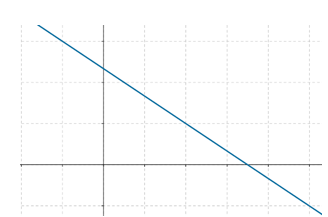Now, let us take a point in the upper half-plane, and the point B which lies vertically below it on the line, as shown: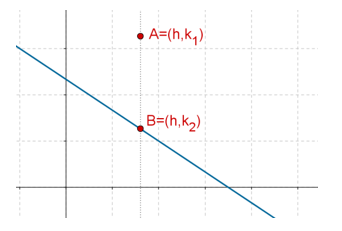Note that since A and are vertically aligned, they will have the same x coordinates. Let’s assume the coordinates of these two points as follows:

$A \equiv \left({h,\;{k_1}} \right),\,\,\,B \equiv \left( {h,\;{k_2}} \right)$

We note that the coordinates of the point B must satisfy the equation of the line, since it lies on the line:

${k_2} = mh + b$

We further note that the coordinate of A must be greater than the y coordinate of B, since A lies above B. Thus, we can say that

${k_1} > {k_2}$

This further means that

${k_1}> mh + b$

We therefore note that every point which lies in the upper half-plane will satisfy the linear inequation

$y > mx + b$

Similarly, every point lying in the lower half-plane will satisfy the linear inequation

$y < mx + b$

And of course, any point lying exactly on the line will satisfy the linear equation

$y = mx + b$

We note the following two special cases:

### (1) Horizontal Line

If a line is horizontal, we have seen that it will have an equation of the form $$y= k,$$ where k is a constant. In this case, the upper half-plane will correspond to the inequation $$y > k,$$ while the lower half-plane will correspond to the inequation $$y < k$$. This is shown in the following figure: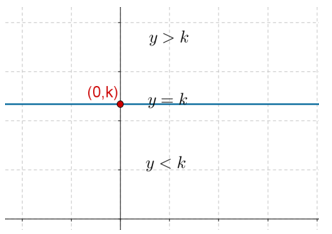### (2) Vertical Line

If a line is vertical, we have seen that it will have an equation of the form $$x= k$$ where k is a constant. In this case, the right half-plane will correspond the inequation $$x > k$$, while the left half-plane will correspond to the inequation $$x < k$$. This is shown in the following figure: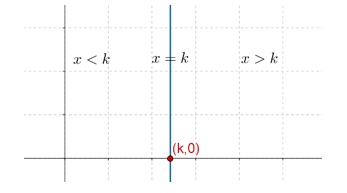Example 1: Plot the graph of the line $$x - 2y = 1$$, and find out the inequations corresponding to the upper and lower half-planes.

Solution: First, we find out any two points on the line and draw the graph:

$\left.\begin{array}{l}x = 1 & \Rightarrow \;\;\;y = 0\\x = - 1 & \Rightarrow \;\;\;y = - 1\end{array}\right\}\,\,\,\,\,\begin{array}{*{20}{c}}{A\left( {1,\;0} \right)}\\{B\left( {- 1,\; - 1} \right)}\end{array}$

The graph is plotted below: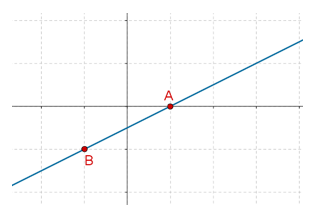To determine the inequation corresponding to the upper half-plane, we write the equation of the line as follows:

$y = \frac{1}{2}x -\frac{1}{2}$

Now, any point lying in the upperhalf-plane will satisfy

\begin{align}&y> \frac{1}{2}x - \frac{1}{2}\\& \Rightarrow \;\;\;2y > x - 1\\& \Rightarrow\;\;\;x - 2y < 1\end{align}

On the other hand, any point lying in the lower half-plane will satisfy

\begin{align}&y< \frac{1}{2}x - \frac{1}{2}\\& \Rightarrow \;\;\;2y < x - 1\\& \Rightarrow\;\;\;x - 2y > 1\end{align}

The following diagram summarizes these facts: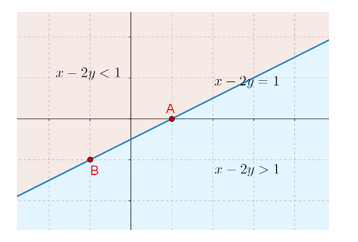Example 2: Plot the half-plane corresponding to the following linear inequation:

$3x - 4y < 5$

Solution: We first write this inequation as follows:

\begin{align}&4y> 3x - 5\\& \Rightarrow \;\;\;y > \frac{3}{4}x - \frac{5}{4} &...\;(*)\end{align}

Now, we convert this inequation to an equation:

$y = \frac{3}{4}x -\frac{5}{4}$

Next, we plot the line corresponding to this equation. For that, we find out two points on the line:

\left.\begin{align}&x = 0\;\; \Rightarrow \;\;y = - \frac{5}{4}\\&x = 3\;\;\Rightarrow \;\;y = 1\end{align} \right\}\begin{array}{*{20}{c}}{A\left( {0,\;- \frac{5}{4}} \right)}\\\!\!\!\!\!\!\!\!\!{B\left( {3,\;1} \right)}\end{array}

The plot of the line is shown below: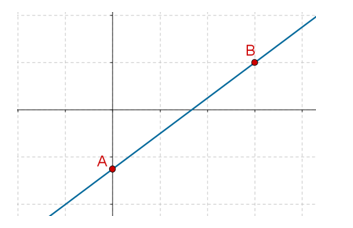Finally, the starred inequation will correspond to the upper half-plane, as shown below: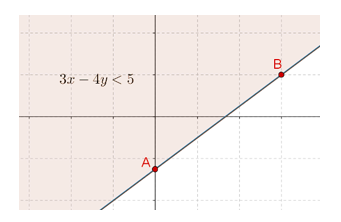Learn math from the experts and clarify doubts instantly

• Instant doubt clearing (live one on one)
• Learn from India’s best math teachers
• Completely personalized curriculum